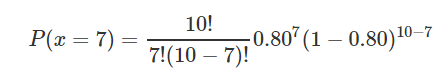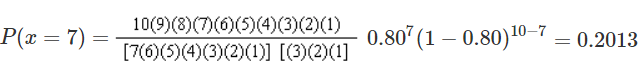# Examples of Binomial Distribution Problems and Solutions

As in any other statistical areas, the understanding of binomial probability comes with exploring binomial distribution examples, problems, answers, and solutions from the real life.

It is not too much to say that the path of mastering statistics and data science starts with probability. And the binomial concept has its core role when it comes to defining the probability of success or failure in an experiment or survey.

On this page you will learn:

• Binomial distribution definition and formula.
• Conditions for using the formula.
• 3 examples of the binomial distribution problems and solutions.

Many real life and business situations are a pass-fail type. For example, if you flip a coin, you either get heads or tails. You either will win or lose a backgammon game. There are only two possible outcomes – success and failure, win and lose.

And the key element here also is that likelihood of the two outcomes may or may not be the same.

So, what is binomial distribution?

Let's define it:

In simple words, a binomial distribution is the probability of a success or failure results in an experiment that is repeated a few or many times.

The prefix “bi” means two. We have only 2 possible incomes.

Binomial probability distributions are very useful in a wide range of problems, experiments, and surveys. However, how to know when to use them?

Let's see the necessary conditions and criteria to use binomial distributions:
• Rule 1: Situation where there are only two possible mutually exclusive outcomes (for example, yes/no survey questions).
• Rule2: A fixed number of repeated experiments and trials are conducted (the process must have a clearly defined number of trials).
• Rule 3: All trials are identical and independent (identical means every trial must be performed the same way as the others; independent means that the result of one trial does not affect the results of the other subsequent trials).
• Rule: 4: The probability of success is the same in every one of the trials.

Notations for Binomial Distribution and the Mass Formula:Where:

• P is the probability of success on any trail.
• q = 1- P – the probability of failure
• n – the number of trails/experiments
• x – the number of successes, it can take the values 0, 1, 2, 3, . . . n.
• nCx = n!/x!(n-x) and denotes the number of combinations of n elements taken x at a time.

Assuming what the nCx means, we can write the above formula in this way:Just to remind that the ! symbol after a number means it’s a factorial. The factorial of a non-negative integer x is denoted by x!. And x! is the product of all positive integers less than or equal to x. For example, 4! = 4 x 3 x 2 x 1 = 24.

Examples of binomial distribution problems:

• The number of defective/non-defective products in a production run.
• Yes/No Survey (such as asking 150 people if they watch ABC news).
• Vote counts for a candidate in an election.
• The number of successful sales calls.
• The number of male/female workers in a company

So, as we have the basis let’s see some binominal distribution examples, problems, and solutions from the real life.

Example 1:

Let’s say that 80% of all business startups in the IT industry report that they generate a profit in their first year. If a sample of 10 new IT business startups is selected, find the probability that exactly seven will generate a profit in their first year.

First, do we satisfy the conditions of the binomial distribution model?

• There are only two possible mutually exclusive outcomes – to generate a profit in the first year or not (yes or no).
• There are a fixed number of trails (startups) – 10.
• The IT startups are independent and it is reasonable to assume that this is true.
• The probability of success for each startup is 0.8.

We know that:

n = 10, p=0.80, q=0.20, x=7

The probability of 7 IT startups to generate a profit in their first year is:This is equivalent to:Interpretation/solution: There is a 20.13% probability that exactly 7 of 10 IT startups will generate a profit in their first year when the probability of profit in the first year for each startup is 80%.

And as we live in the internet ERA and there are so many online calculators available for free use, there is no need to calculate by hand.

Just use one of the online calculators for binomial distribution (for example this one).

The important points here are to know when to use the binomial formula and to know what are the values of p, q, n, and x.

Also, binomial probabilities can be computed in an Excel spreadsheet using the =BINOMDIST function.

Example 2:

Your basketball team is playing a series of 5 games against your opponent. The winner is those who wins more games (out of 5).

Let assume that your team is much more skilled and has 75% chances of winning. It means there is a 25% chance of losing.

What is the probability of your team get 3 wins?

We need to find out.

In this example:

n = 5, p=0.75, q=0.25, x=3

Let’s replace in the formula to get the answer:Interpretation: the probability that you win 3 games is 0.264.

Example 3:

A box of candies has many different colors in it. There is a 15% chance of getting a pink candy. What is the probability that exactly 4 candies in a box are pink out of 10?

We have that:

n = 10, p=0.15, q=0.85, x=4

When we replace in the formula:Interpretation: The probability that exactly 4 candies in a box are pink is 0.04.

The above binomial distribution examples aim to help you understand better the whole idea of binomial probability.

If you need more examples in statistics and data science area, our posts descriptive statistics examples and categorical data examples might be useful for you.